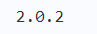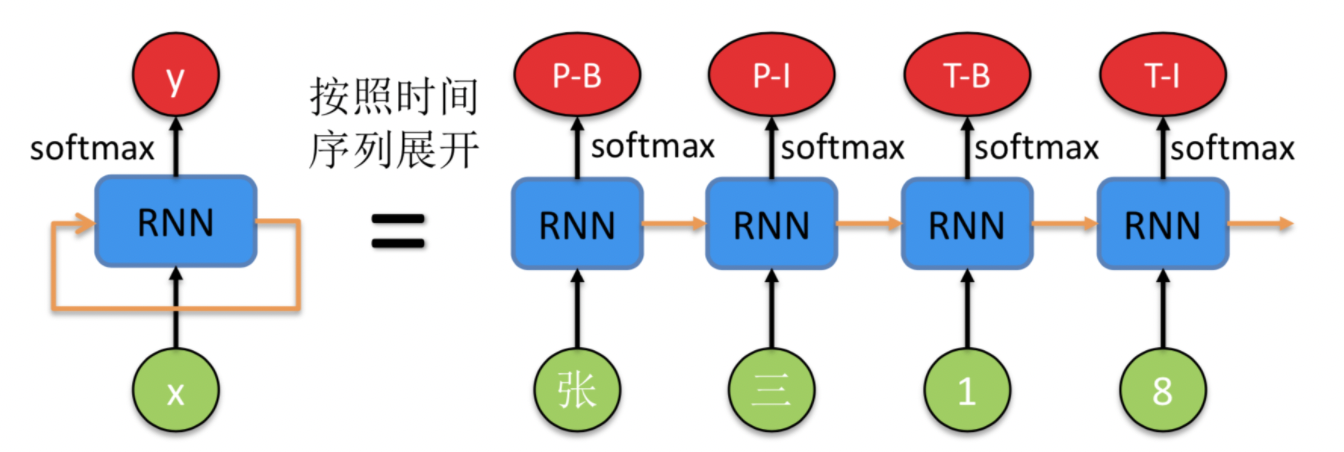# 【自然语言处理（NLP）】基于LSTM的命名实体识别(进阶).

.

.

.# 前言

## (二)、环境配置

import paddle
import numpy as np
import matplotlib.pyplot as plt# 一、优化进阶-使用预训练的词向量优化模型效果

## (一)、导入相关包

from paddlenlp.embeddings import TokenEmbedding # EMB

del model
del preds
del network


## (二)、定义BiGRUWithCRF2

class BiGRUWithCRF2(nn.Layer):
def __init__(self,
emb_size,
hidden_size,
word_num,
label_num,
use_w2v_emb=True):
super(BiGRUWithCRF2, self).__init__()
if use_w2v_emb:
self.word_emb = TokenEmbedding(
extended_vocab_path='./data/word.dic', unknown_token='OOV')
else:
self.word_emb = nn.Embedding(word_num, emb_size)
self.gru = nn.GRU(emb_size,
hidden_size,
num_layers=2,
direction='bidirectional')
self.fc = nn.Linear(hidden_size * 2, label_num + 2)  # BOS EOS
self.crf = LinearChainCrf(label_num)
self.decoder = ViterbiDecoder(self.crf.transitions)

def forward(self, x, lens):
embs = self.word_emb(x)
output, _ = self.gru(embs)
output = self.fc(output)
_, pred = self.decoder(output, lens)
return output, lens, pred


## (三)、模型构建

network = BiGRUWithCRF2(300, 300, len(word_vocab), len(label_vocab))
crf_loss = LinearChainCrfLoss(network.crf)
chunk_evaluator = ChunkEvaluator(label_list=label_vocab.keys(), suffix=True)
model.prepare(optimizer, crf_loss, chunk_evaluator)


model.fit(train_data=train_loader,
epochs=10,
save_dir='./results',
log_freq=1)


model.evaluate(eval_data=test_loader)



## (四)、模型验证

outputs, lens, decodes = model.predict(test_data=test_loader)
preds = parse_decodes(test_ds, decodes, lens, label_vocab)

print('\n'.join(preds[:5]))


# 二、概念解释

## (一)、门控循环单元GRU

BIGRU是一种经典的循环神经网络（RNN，Recurrent Neural Network），前面一些步骤基本是把该模型当做是黑盒子来用，这里我们重点解释下其概念和相关原理。一个 RNN 的示意图如下所示：GRU为了解决长期记忆和反向传播中梯度问题而提出来的，和LSTM一样能够有效对长序列建模，且GRU训练效率更高。

## (二)、条件随机场CRFCRF 本质是一个无向图，其中绿色点表示输入，红色点表示输出。点与点之间的边可以分成两类，一类是 x x y y 之间的连线，表示其相关性；另一类是相邻时刻的 y y 之间的相关性。也就是说，在预测某时刻 y y 时，同时要考虑相邻的标签解决。当 CRF 模型收敛时，就会学到类似 P-B 和 T-I 作为相邻标签的概率非常低。

# 总结

ps：更多精彩内容还请进入本文专栏人工智能，进行查看，欢迎大家支持与指教啊～(￣▽￣～)~### 网站公告